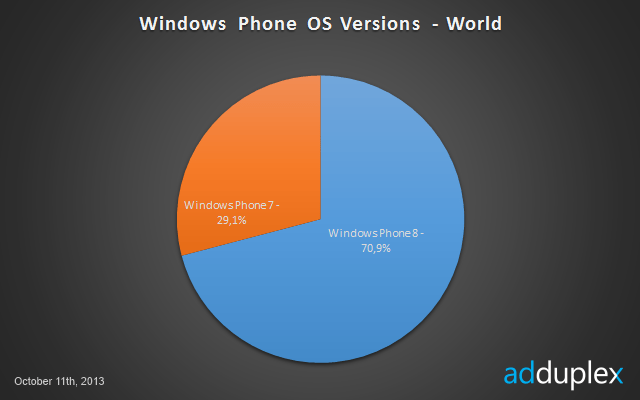[embedit snippet=”fluids”]

September stats:On popular demand, we try to predict what Windows Phone volume could have been sold in Q3. This calculation is based on Adduplex stats. Assuming 25 + 7 = 32 million active Windows Phones till the end of Q2, we see that WP8 has grown further by 10% in Q3 from 61% in June to 71% in September.

Let us assume conservatively that only 0.8 million WP7 devices were sold in Q2. Then considering 40% and 45% skew in Adduplex data in start and end of Q3, we will have ((32*0.39*1.4)+0.8) / (0.29*1.45) = 43.5 million devices at end of Q3.

Hence WP devices sold in Q3 = 43.5-32 =11.5 million devices. Nokia may have sold 11.5 * 0.85 = 9.7 million Lumia devices.

Disclaimer: This calculation is based on “Adduplex stats” and may vary from actual installed base figures as the apps usage by newer devices is always higher as compared to older devices. Though we use coefficients to correct the skew, but still this calculation is for academic interest only!!